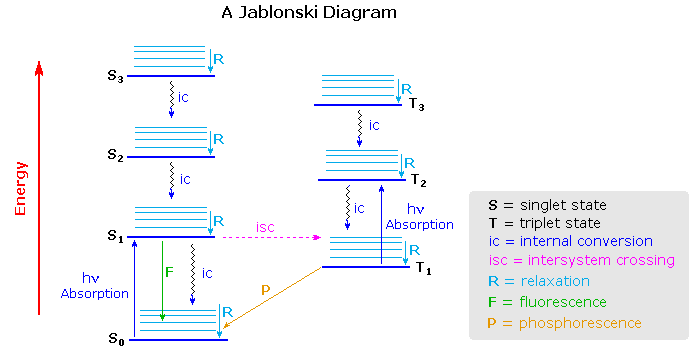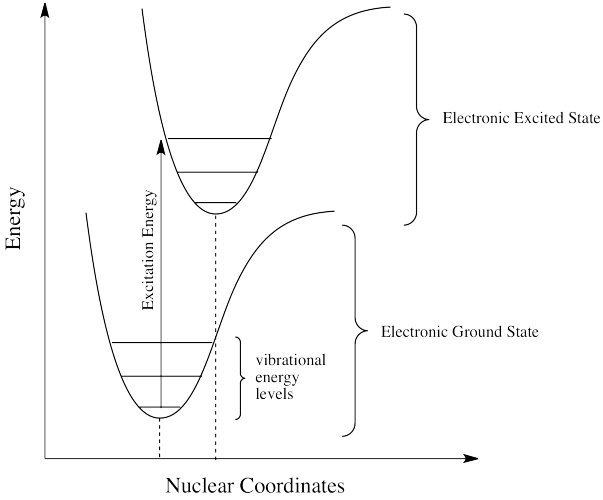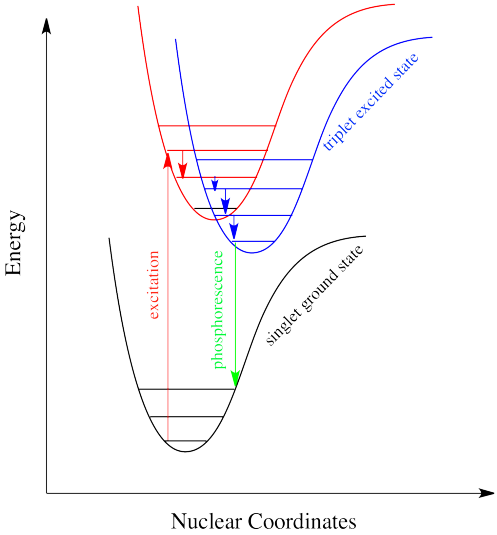# 14.7: Fluorescence and Phosphorescence

$$\newcommand{\vecs}{\overset { \rightharpoonup} {\mathbf{#1}} }$$ $$\newcommand{\vecd}{\overset{-\!-\!\rightharpoonup}{\vphantom{a}\smash {#1}}}$$$$\newcommand{\id}{\mathrm{id}}$$ $$\newcommand{\Span}{\mathrm{span}}$$ $$\newcommand{\kernel}{\mathrm{null}\,}$$ $$\newcommand{\range}{\mathrm{range}\,}$$ $$\newcommand{\RealPart}{\mathrm{Re}}$$ $$\newcommand{\ImaginaryPart}{\mathrm{Im}}$$ $$\newcommand{\Argument}{\mathrm{Arg}}$$ $$\newcommand{\norm}{\| #1 \|}$$ $$\newcommand{\inner}{\langle #1, #2 \rangle}$$ $$\newcommand{\Span}{\mathrm{span}}$$ $$\newcommand{\id}{\mathrm{id}}$$ $$\newcommand{\Span}{\mathrm{span}}$$ $$\newcommand{\kernel}{\mathrm{null}\,}$$ $$\newcommand{\range}{\mathrm{range}\,}$$ $$\newcommand{\RealPart}{\mathrm{Re}}$$ $$\newcommand{\ImaginaryPart}{\mathrm{Im}}$$ $$\newcommand{\Argument}{\mathrm{Arg}}$$ $$\newcommand{\norm}{\| #1 \|}$$ $$\newcommand{\inner}{\langle #1, #2 \rangle}$$ $$\newcommand{\Span}{\mathrm{span}}$$$$\newcommand{\AA}{\unicode[.8,0]{x212B}}$$

## Singlet and Triplet Excited State

Understanding the difference between fluorescence and phosphorescence requires the knowledge of electron spin and the differences between singlet and triplet states. The Pauli Exclusion principle states that two electrons in an atom cannot have the same four quantum numbers ($$n$$, $$l$$, $$m_l$$, $$m_s$$) and only two electrons can occupy each orbital where they must have opposite spin states. These opposite spin states are called spin pairing. Because of this spin pairing, most molecules do not exhibit a magnetic field and are diamagnetic. In diamagnetic molecules, electrons are not attracted or repelled by the static electric field. Free radicals are paramagnetic because they contain unpaired electrons have magnetic moments that are attracted to the magnetic field.

Singlet state is defined when all the electron spins are paired in the molecular electronic state and the electronic energy levels do not split when the molecule is exposed into a magnetic field. A doublet state occurs when there is an unpaired electron that gives two possible orientations when exposed in a magnetic field and imparts different energy to the system. A singlet or a triplet can form when one electron is excited to a higher energy level. In an excited singlet state, the electron is promoted in the same spin orientation as it was in the ground state (paired). In a triplet excited stated, the electron that is promoted has the same spin orientation (parallel) to the other unpaired electron. The difference between the spins of ground singlet, excited singlet, and excited triplet is shown in Figure $$\PageIndex{1}$$. Singlet, doublet and triplet is derived using the equation for multiplicity, 2S+1, where S is the total spin angular momentum (sum of all the electron spins). Individual spins are denoted as spin up (s = +1/2) or spin down (s = -1/2). If we were to calculated the S for the excited singlet state, the equation would be 2(+1/2 + -1/2)+1 = 2(0)+1 = 1, therefore making the center orbital in the figure a singlet state. If the spin multiplicity for the excited triplet state was calculated, we obtain 2(+1/2 + +1/2)+1 = 2(1)+1 =3, which gives a triplet state as expected.Figure $$\PageIndex{1}$$: Spin in the ground and excited states

The difference between a molecule in the ground and excited state is that the electrons is diamagnetic in the ground state and paramagnetic in the triplet state.This difference in spin state makes the transition from singlet to triplet (or triplet to singlet) more improbable than the singlet-to-singlet transitions. This singlet to triplet (or reverse) transition involves a change in electronic state. For this reason, the lifetime of the triplet state is longer the singlet state by approximately 104 seconds fold difference.The radiation that induced the transition from ground to excited triplet state has a low probability of occurring, thus their absorption bands are less intense than singlet-singlet state absorption. The excited triplet state can be populated from the excited singlet state of certain molecules which results in phosphorescence. These spin multiplicities in ground and excited states can be used to explain transition in photoluminescence molecules by the Jablonski diagram.

## Jablonski Diagrams

The Jablonski diagram that drawn below is a partial energy diagram that represents the energy of photoluminescent molecule in its different energy states. The lowest and darkest horizontal line represents the ground-state electronic energy of the molecule which is the singlet state labeled as $$S_o$$. At room temperature, majority of the molecules in a solution are in this state.Figure $$\PageIndex{2}$$: Partial Jablonski Diagram for Absorption, Fluorescence, and Phosphorescence. from Bill Reusch.

The upper lines represent the energy state of the three excited electronic states: S1and S2 represent the electronic singlet state (left) and T1 represents the first electronic triplet state (right). The upper darkest line represents the ground vibrational state of the three excited electronic state.The energy of the triplet state is lower than the energy of the corresponding singlet state.

There are numerous vibrational levels that can be associated with each electronic state as denoted by the thinner lines. Absorption transitions (blues lines in Figure $$\PageIndex{2}$$) can occur from the ground singlet electronic state (So) to various vibrational levels in the singlet excited vibrational states. It is unlikely that a transition from the ground singlet electronic state to the triplet electronic state because the electron spin is parallel to the spin in its ground state (Figure $$\PageIndex{1}$$). This transition leads to a change in multiplicity and thus has a low probability of occurring which is a forbidden transition. Molecules also go through vibration relaxation to lose any excess vibrational energy that remains when excited to the electronic states ($$S_1$$ and $$S_2$$) as demonstrated in wavy lines in Figure $$\PageIndex{2}$$. The knowledge of forbidden transition is used to explain and compare the peaks of absorption and emission.

## Relaxation and Fluorescence

Sometimes, when an excited state species relaxes, giving off a photon, the wavelength of the photon is different from the one that initially led to excitation. When this happens, the photon is invariably red-shifted; its wavelength is longer than the initial one. This situation is called "fluorescence".How can that be? Isn't energy quantized? How is the molecule suddenly taking a commission out of the energy the original photon brought with it? This discrepancy is related to the Franck-Condon principle from the previous page. When an electron is promoted to an electronic excited state, it often ends up in an excited vibrational state as well. Thus, some of the energy put into electronic excitation is immediately passed into vibrational energy. Vibrational energy, however, doesn't just travel in photons. It can be gained or lost through molecular collisions and heat transfer.The electron might simply drop down again immediately; a photon would be emitted of exactly the same wavelength as the one that was previously absorbed. On the other hand, if the molecule relaxes into a lower vibrational state, some of that initial energy will have been lost as heat. When the electron relaxes, the distance back to the ground state is a little shorter. The photon that is emitted will have lower energy and longer wavelength than the initial one.Just how does a molecule undergo vibrational relaxation? Vibrational energy is the energy used to lengthen or shorten bonds, or to widen or squeeze bond angles. Given a big enough molecule, some of this vibrational energy could be transferred into bond lengths and angles further away from the electronic transition. Otherwise, if the molecule is small, it may transfer some of its energy in collisions with other molecules.

Note

There are many examples of energy being transferred this way in everyday life. In a game of pool, one billiard ball can transfer its energy to another, sending it toward the pocket. Barry Bonds can transfer a considerable amount of energy through his bat into a baseball, sending it out of the park, just as Serena Williams can send a whole lot of energy whizzing back at her sister.

Exercise 1

How does the energy of an electronic absorption compare to other processes? To find out, you might consider the excitation of an entire mole of molecules, rather than a sinle molecule absorbing a single photon. Calculate the energy in kJ/mol for the following transitions.

1. absorbance at 180 nm (ultraviolet)
2. absorbance at 476 nm (blue)
3. absorbance at 645 nm (red)

Exercise 2

How does the energy of an excitation between vibrational states compare to that of an electronic excitation? Typically, infrared absorptions are reported in cm-1, which is simply what it looks like: the reciprocal of the wavelength in cm. Because wavelength and frequency are inversely related, wavenumbers are considered a frequency unit. Calculate the energy in kJ/mol for the following transitions.

1. absorbance at 3105 cm-1
2. absorbance at 1695 cm-1
3. absorbance at 963 cm-1

In molecules, as one molecule drops to a lower vibrational state, the other will hop up to a higher vibrational state with the energy it gains. In the drawing below, the red molecule is in an electronic excited and vibrational state. In a collision, it transfers some of its vibrational energy to the blue molecule.If electrons can get to a lower energy state, and give off a little energy at a time, by hopping down to lower and lower vibrational levels, do they need to give off a giant photon at all? Maybe they can relax all the way down to the ground state via vibrational relaxation. That is certainly the case. Given lots of vibrational energy levels, and an excited state that is low enough in energy so that some of its lower vibrational levels overlap with some of the higher vibrational levels of the ground state, the electron can hop over from one state to the other, without releasing a photon.This event is called a "radiationless transition", because it occurs without release of a photon. The electron simply slides over from a low vibrational state of the excited electronic state to a high vibrational state of the electronic ground state. If the electron simply keeps dropping a vibrational level at a time back to the ground state, the process is called "internal conversion".

Internal conversion has an important consequence. Because the absorption of UV and visible light can result in energy transfer into vibrational states, much of the energy that is absorbed from these sources is converted into heat. That can be a good thing if you happen to be a marine iguana trying to warm up in the sun after a plunge in the icy Pacific. It can also be a tricky thing if you are a process chemist trying to scale up a photochemical reaction for commercial production of a pharmaceutical, because you have to make sure the system has adequate cooling available.

There is a very similar event, called "intersystem crossing", that leads to the electron getting caught between the excited state and the ground state. Just as, little by little, vibrational relaxation can lead the electron back onto the ground state energy surface, it can also lead the electron into states that are intermediate in energy.

For example, suppose an organic molecule undergoes electronic excitation. Generally, organic molecules have no unpaired electrons. Their ground states are singlet states. According to one of our selection rules for electronic excitation, the excited state must also have no unpaired electrons. In other words, the spin on the electron that gets excited is the same after excitation as it was before excitation.

However, that's not the lowest possible energy state for that electron. When we think about atomic orbital filling, there is a rule that governs the spin on the electrons in degenerate orbitals: in the lowest energy state, spin is maximized (Hund's rule). In other words, when we draw a picture of the valence electron configuration of nitrogen, we show nitrogen's three p electrons each in its own orbital, with their spins parallel.The picture with three unpaired electrons, all with parallel spins, shows a nitrogen in the quartet spin state. Having one of those spins point the other way would result in a different spin state. One pair of electrons in the p level would be spin-paired, one up and one down, even though they are in different p orbitals. That would leave one electron without an opposite partner. The nitrogen would be in a doublet spin state. That is not what happens. The spin state on the left is lower in energy than the state on the right. That's just one of the rules of quantum mechanics (Hund's rule): maximize spin when orbitals are singly occupied.

It's the same in a molecule with the triplet state lower in energy than the singlet state. Why didn't the electron get excited to the triplet state in the first place? That's against the rules. But sliding down vibrationally onto the triplet state from the singlet excited state is not, because it doesn't involve absorption of a photon.Intersystem crossing can have important consequences in reaction chemistry because it allows access to triplet states that are not normally avaiable in many molecules. Because triplet states feature unpaired electrons, their reactivity is often typified by radical processes. That means an added suite of reactions can be accessed via this process.

## Phosphorescence: A Radiationless Transition Followed by Emission

Intersystem crossing is one way a system can end up in a triplet excited state. Even though this state is lower in energy than a singlet excited state, it cannot be accessed directly via electronic excitation because that would violate the spin selection rule (\Delta S=0\). That's where the electron gets stuck, though. The quick way back down to the bottom is by emitting a photon, but because that would involve a change in spin state, it is not allowed. Realistically speaking, that means it takes a long time. By "a long time", we might mean a few seconds, several minutes, or possibly even hours. Eventually, the electron can drop back down, accompanied by the emission of a photon. This situation is called "phosphorescence".Molecules that display phosphorescence are often incorporated into toys and shirts so that they will glow in the dark.